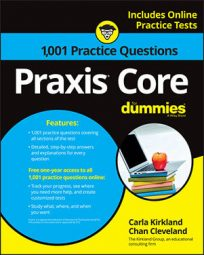##### Praxis Core: 1,001 Practice Questions For DummiesAcronyms are wonderful things—especially when it comes to remembering order of operations. In fact, the acronym GEMDAS will definitely serve you well when you take the Praxis Core exam.

GEMDAS (Grouping, Exponents, Multiplication, Division, Addition, and Subtraction) is equivalent to PEMDAS. The only difference is that P stands for parentheses instead of grouping.

The following practice questions will test your ability to apply GEMDAS in order to solve different expressions. (Hint: for the first question, remember that a fraction bar separates the numerator and denominator into two groups!)

## Practice questions

1. What is the value of this expression?

2. Evaluate this expression.

1. The correct answer is Choice (B). Follow the order of operations by using GEMDAS. Keep in mind that a fraction bar is a grouping symbol. It groups the numerator and also groups the denominator.

2. The correct answer is Choice (C).

You can use your calculator for this problem, putting fractions in parentheses and using the division sign in place of a fraction bar. Otherwise, follow the order of operations by using GEMDAS: